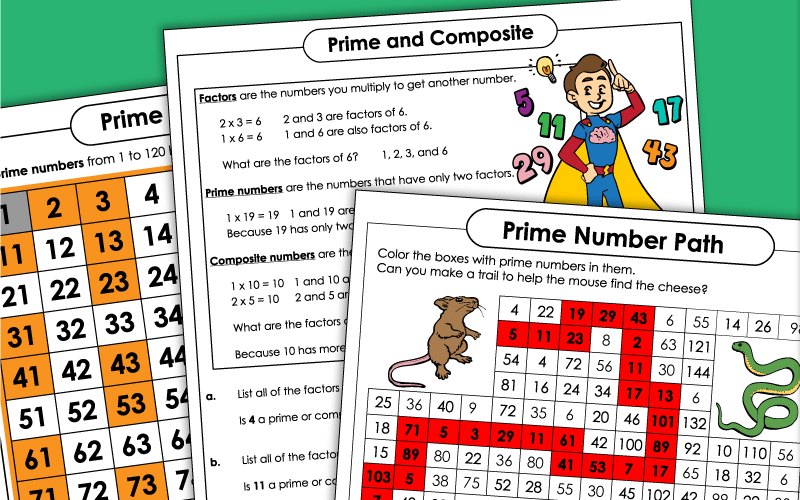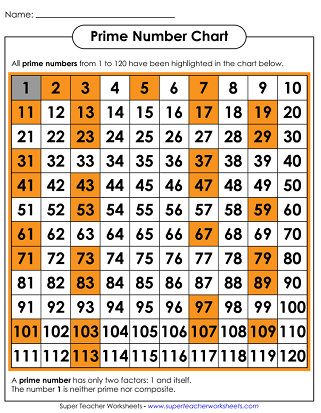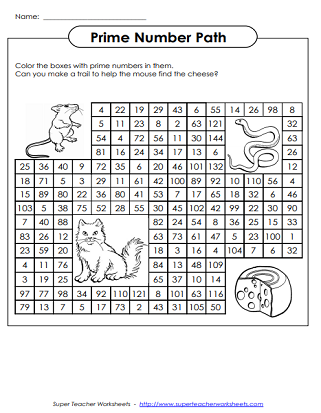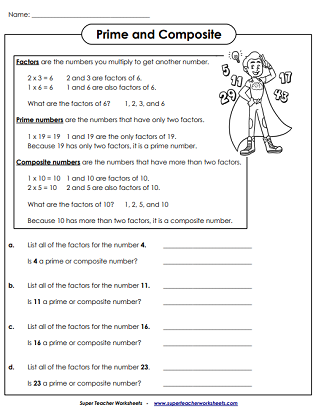# Prime and Composite Numbers

Use these printable PDF worksheets to teach students to find and identify prime and composite numbers.

For worksheets on prime factorization, please jump over to our
factoring worksheets section.## Prime & Composite

This worksheet is a basic introduction to finding and identifying prime and composite numbers.
On this printable worksheet, your students list all of the factors of a given number. Then they tell whether the number is composite or prime.
Students will list factors of a number on the table. Then they will tell which numbers are prime and which are composite.
Help the mouse find his way to the block of cheese. To do so, students must make a path by coloring only the boxes with prime numbers.

## Prime Number Chart

This 120 chart highlights prime numbers in orange.
Use colored pencils or crayons to shade all of the prime numbers in red and the composite numbers in blue.

## Sieve of Eratosthenes

Use the sieve of Eratosthenes is a number table that you can use to find prime numbers. Students use colored pencils to mark the multiples of 2, 3, 5, and 7. The numbers that are not marked are prime numbers.

Factoring Worksheets

This page contains prime factorization trees, as well as activities that require students to list factors of a number. There are also worksheets on proper factors and perfect numbers.

Multiples

Here are some activities to help students find multiples of numbers.

## Pictures of WorksheetsMy Account
Site Information